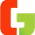## How to solve brain busters?

Brain busters are tricky in nature & can be solved with IQ. These questions aren’t straight forward & would need your wits & brain power. In this lesson we are going to find the best approach to solve 2 types of brain busters.

The best way to solve brain teasers is to see whether it requires logic or needs initial guess & solve accordingly. Some brain busters can be solved by putting smaller value of x & incrementing x by any number, & solving again until you find the answer. You can guess an initial value of x & check the nature of answer. Initial guess is very important because it can save time in finding solution.

How to solve brain teasers?

For example:

We want to find 2^x = 8

Now we don't know the value of x but we can suppose that value of x is 1

We can solve this question by putting x = 1

2^1 = 2

Hence answer is not equal to R.H.S. so we increment x by 1. Hence putting x = 2

2^2 = 4

Again we haven’t reach the answer but we are getting closer. We can predict that if we put a greater value of x then we can solve this question.

Now putting x = 3 & solving:

2^3 = 8

Hence we have found the solution.

Now see other types of brain busters that are logical in nature. Here you need to find a pattern & solve questions by using the same pattern throughout the problem.

For example:

If
2 = 12
3 = 10
4 = 8

Then
5 = ?

Now it is clear that this question is incorrect. But there is a supposition (if) which means it is logical.

First we need to find pattern.
2 = 12

When we increment 2 by 1, we decrement 12 by 2
2 + 1 = 12 – 2
3 = 10

Hence it is equal to next equation

Now using the same pattern:
3 + 1 = 10 – 2
4 = 8

It is again equal to next equation. Hence this pattern is correct.

Now finding the unknown:
4 + 1 = 8 – 2
5 = 6

### Summary:

Hopefully you have learned how to solve two types of brain busters. One that allows you to make initial guess by seeing the nature of question & then make increment / decrement to reach the answer. Another type of brain teaser uses logic to find the answer. In this type of questions, you need to find pattern that holds true in the full problem.

1.solved my prblem using your method. Thank

1.Thanks a lot for a positive feedback. Every comment means a lot to us & thank you so much for your kind words.

2.Good work

1.